# Reproducing Goeyvaerts et al. (2018) using ergm.multi

#### ergm.multi version 0.2.0 (2023-05-29)

library(ergm.multi)
library(dplyr)
library(purrr)
library(tibble)
library(ggplot2)

# Obtaining data

The list of networks studied by Goeyvaerts et al. (2018) is included in this package:

data(Goeyvaerts)
length(Goeyvaerts)
##  318

An explanation of the networks, including a list of their network (%n%) and vertex (%v%) attributes, can be obtained via ?Goeyvaerts. A total of 318 complete networks were collected, then two were excluded due to “nonstandard” family composition:

Goeyvaerts %>% discard(%n%, "included") %>% map(as_tibble, unit="vertices")
## []
## # A tibble: 4 × 5
##   vertex.names   age gender na    role
##          <int> <int> <chr>  <lgl> <chr>
## 1            1    32 F      FALSE Mother
## 2            2    48 F      FALSE Grandmother
## 3            3    32 M      FALSE Father
## 4            4    10 F      FALSE Child
##
## []
## # A tibble: 3 × 5
##   vertex.names   age gender na    role
##          <int> <int> <chr>  <lgl> <chr>
## 1            1    29 F      FALSE Mother
## 2            2    28 F      FALSE Mother
## 3            3     0 F      FALSE Child

To reproduce the analysis, exclude them as well:

G <- Goeyvaerts %>% keep(%n%, "included")

# Data summaries

Obtain weekday indicator, network size, and density for each network, and summarize them as in Goeyvaerts et al. (2018) Table 1:

G %>% map(~list(weekday = . %n% "weekday",
n = network.size(.),
d = network.density(.))) %>% bind_rows() %>%
group_by(weekday, n = cut(n, c(1,2,3,4,5,9))) %>%
summarize(nnets = n(), p1 = mean(d==1), m = mean(d)) %>% kable()
weekday n nnets p1 m
FALSE (1,2] 3 1.0000000 1.0000000
FALSE (2,3] 19 0.7368421 0.8771930
FALSE (3,4] 48 0.8541667 0.9618056
FALSE (4,5] 18 0.7777778 0.9500000
FALSE (5,9] 3 1.0000000 1.0000000
TRUE (1,2] 9 1.0000000 1.0000000
TRUE (2,3] 53 0.9056604 0.9622642
TRUE (3,4] 111 0.7747748 0.9279279
TRUE (4,5] 39 0.6410256 0.8974359
TRUE (5,9] 13 0.4615385 0.8454212

# Reproducing ERGM fits

We now reproduce the ERGM fits. First, we extract the weekday networks:

G.wd <- G %>% keep(%n%, "weekday")
length(G.wd)
##  225

Next, we specify the multi-network model using the N(formula, lm) operator. This operator will evaluate the ergm formula formula on each network, weighted by the predictors passed in the one-sided lm formula, which is interpreted the same way as that passed to the built-in lm() function, with its “data” being the table of network attributes.

Since different networks may have different compositions, to have a consistent model, we specify a consistent list of family roles.

roleset <- sort(unique(unlist(lapply(G.wd, %v%, "role"))))

We now construct the formula object, which will be passed directly to ergm():

# Networks() function tells ergm() to model these networks jointly.
f.wd <- Networks(G.wd) ~
# This N() operator adds three edge counts:
N(~edges,
~ # one total for all networks  (intercept implicit as in lm),
I(n<=3)+ # one total for only small households, and
I(n>=5) # one total for only large households.
) +

# This N() construct evaluates each of its terms on each network,
# then sums each statistic over the networks:
N(
# First, mixing statistics among household roles, including only
# father-mother, father-child, and mother-child counts.
# Since tail < head in an undirected network, in the
# levels2 specification, it is important that tail levels (rows)
# come before head levels (columns). In this case, since
# "Child" < "Father" < "Mother" in alphabetical order, the
# row= and col= categories must be sorted accordingly.
~mm("role", levels = I(roleset),
levels2=~.%in%list(list(row="Father",col="Mother"),
list(row="Child",col="Father"),
list(row="Child",col="Mother"))) +
# Second, the nodal covariate effect of age, but only for
# edges between children.
F(~nodecov("age"), ~nodematch("role", levels=I("Child"))) +
# Third, 2-stars.
kstar(2)
) +

# This N() adds one triangle count, totalled over all households
# with at least 6 members.
N(~triangles, ~I(n>=6))

See ergmTerm?mm for documentation on the mm term used above. Now, we can fit the model:

# (Set seed for predictable run time.)
fit.wd <- ergm(f.wd, control=snctrl(seed=123))
summary(fit.wd)
## Call:
## ergm(formula = f.wd, control = snctrl(seed = 123))
##
## Monte Carlo Maximum Likelihood Results:
##
##                                                         Estimate Std. Error MCMC % z value Pr(>|z|)
## N(1)~edges                                               0.86426    0.53992      0   1.601 0.109440
## N(I(n <= 3)TRUE)~edges                                   1.48408    0.44382      0   3.344 0.000826 ***
## N(I(n >= 5)TRUE)~edges                                  -0.79104    0.20414      0  -3.875 0.000107 ***
## N(1)~mm[role=Child,role=Father]                         -0.65385    0.48477      0  -1.349 0.177405
## N(1)~mm[role=Child,role=Mother]                          0.11337    0.52017      0   0.218 0.827466
## N(1)~mm[role=Father,role=Mother]                         0.21252    0.59072      0   0.360 0.719024
## N(1)~F(nodematch("role",levels=I("Child")))~nodecov.age -0.07222    0.01677      0  -4.306  < 1e-04 ***
## N(1)~kstar2                                             -0.25739    0.21293      0  -1.209 0.226731
## N(1)~triangle                                            2.04762    0.31050      0   6.594  < 1e-04 ***
## N(I(n >= 6)TRUE)~triangle                               -0.28208    0.11288      0  -2.499 0.012460 *
## ---
## Signif. codes:  0 '***' 0.001 '**' 0.01 '*' 0.05 '.' 0.1 ' ' 1
##
##      Null Deviance: 1975.5  on 1425  degrees of freedom
##  Residual Deviance:  609.7  on 1415  degrees of freedom
##
## AIC: 629.7  BIC: 682.3  (Smaller is better. MC Std. Err. = 0.6256)

Similarly, we can extract the weekend network, and fit it to a smaller model. We only need one N() operator, since all statistics are applied to the same set of networks, namely, all of them.

G.we <- G %>% discard(%n%, "weekday")
fit.we <- ergm(Networks(G.we) ~
N(~edges +
mm("role", levels=I(roleset),
levels2=~.%in%list(list(row="Father",col="Mother"),
list(row="Child",col="Father"),
list(row="Child",col="Mother"))) +
F(~nodecov("age"), ~nodematch("role", levels=I("Child"))) +
kstar(2) +
triangles), control=snctrl(seed=123))
summary(fit.we)
## Call:
## ergm(formula = Networks(G.we) ~ N(~edges + mm("role", levels = I(roleset),
##     levels2 = ~. %in% list(list(row = "Father", col = "Mother"),
##         list(row = "Child", col = "Father"), list(row = "Child",
##             col = "Mother"))) + F(~nodecov("age"), ~nodematch("role",
##     levels = I("Child"))) + kstar(2) + triangles), control = snctrl(seed = 123))
##
## Monte Carlo Maximum Likelihood Results:
##
##                                                         Estimate Std. Error MCMC % z value Pr(>|z|)
## N(1)~edges                                               2.07548    1.56854      0   1.323  0.18577
## N(1)~mm[role=Child,role=Father]                         -1.13181    1.60122      0  -0.707  0.47966
## N(1)~mm[role=Child,role=Mother]                          0.26025    1.70174      0   0.153  0.87845
## N(1)~mm[role=Father,role=Mother]                        -0.68946    1.66676      0  -0.414  0.67913
## N(1)~F(nodematch("role",levels=I("Child")))~nodecov.age -0.17149    0.05762      0  -2.976  0.00292 **
## N(1)~kstar2                                             -0.86458    0.35552      0  -2.432  0.01502 *
## N(1)~triangle                                            3.56650    0.76602      0   4.656  < 1e-04 ***
## ---
## Signif. codes:  0 '***' 0.001 '**' 0.01 '*' 0.05 '.' 0.1 ' ' 1
##
##      Null Deviance: 802.7  on 579  degrees of freedom
##  Residual Deviance: 132.9  on 572  degrees of freedom
##
## AIC: 146.9  BIC: 177.5  (Smaller is better. MC Std. Err. = 1.075)

# Diagnostics

Perform diagnostic simulation (Krivitsky, Coletti, and Hens 2022), summarize the residuals, and make residuals vs. fitted and scale-location plots:

gof.wd <- gofN(fit.wd, GOF = ~ edges + kstar(2) + triangles)
summary(gof.wd)
## $Observed/Imputed values ## edges kstar2 triangle ## Min. : 1.000 Min. : 0.00 Min. : 0.000 ## 1st Qu.: 3.000 1st Qu.: 3.00 1st Qu.: 1.000 ## Median : 6.000 Median :12.00 Median : 4.000 ## Mean : 5.778 Mean :13.55 Mean : 4.324 ## 3rd Qu.: 6.000 3rd Qu.:12.00 3rd Qu.: 4.000 ## Max. :18.000 Max. :78.00 Max. :23.000 ## NA's :9 NA's :9 ## ##$Fitted values
##      edges            kstar2          triangle
##  Min.   : 0.790   Min.   : 2.610   Min.   : 0.810
##  1st Qu.: 2.960   1st Qu.: 7.923   1st Qu.: 2.350
##  Median : 5.590   Median :10.590   Median : 3.375
##  Mean   : 5.773   Mean   :13.509   Mean   : 4.309
##  3rd Qu.: 5.820   3rd Qu.:11.510   3rd Qu.: 3.770
##  Max.   :14.720   Max.   :57.990   Max.   :19.090
##                   NA's   :9        NA's   :9
##
## $Pearson residuals ## edges kstar2 triangle ## Min. :-4.80557 Min. :-4.07257 Min. :-3.93827 ## 1st Qu.: 0.20310 1st Qu.: 0.19321 1st Qu.: 0.19607 ## Median : 0.36472 Median : 0.38569 Median : 0.40094 ## Mean : 0.01166 Mean : 0.01272 Mean : 0.01938 ## 3rd Qu.: 0.47667 3rd Qu.: 0.50955 3rd Qu.: 0.53504 ## Max. : 1.27066 Max. : 1.47434 Max. : 1.60607 ## NA's :9 NA's :9 ## ##$Variance of Pearson residuals
## $Variance of Pearson residuals$edges
##  1.012602
##
## $Variance of Pearson residuals$kstar2
##  0.9713602
##
## $Variance of Pearson residuals$triangle
##  0.9532192
##
##
## $Std. dev. of Pearson residuals ##$Std. dev. of Pearson residuals$edges ##  1.006281 ## ##$Std. dev. of Pearson residuals$kstar2 ##  0.9855761 ## ##$Std. dev. of Pearson residuals\$triangle
##  0.9763295

Variances of Pearson residuals substantially greater than 1 suggest unaccounted-for heterogeneity.

autoplot(gof.wd)
## []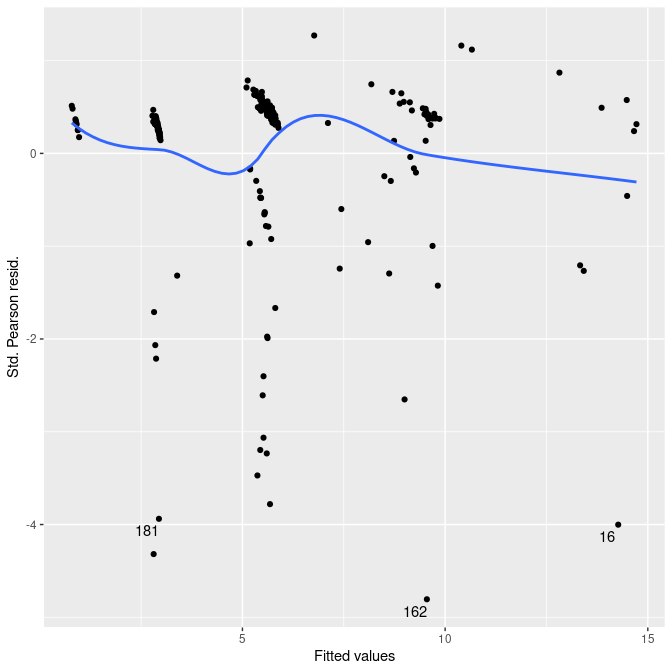##
## []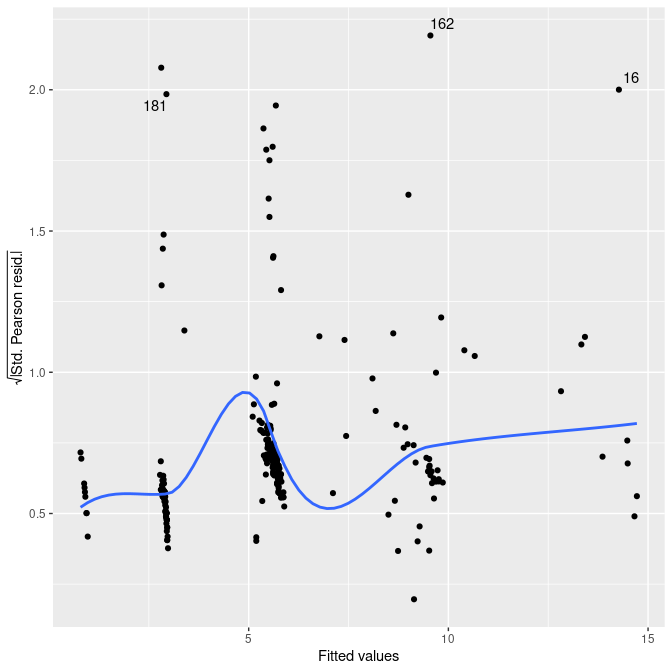##
## []
## Warning: Removed 9 rows containing non-finite values (stat_smooth()).
## Warning: Removed 9 rows containing missing values (geom_point()).
## Warning: Removed 9 rows containing missing values (geom_text_repel()).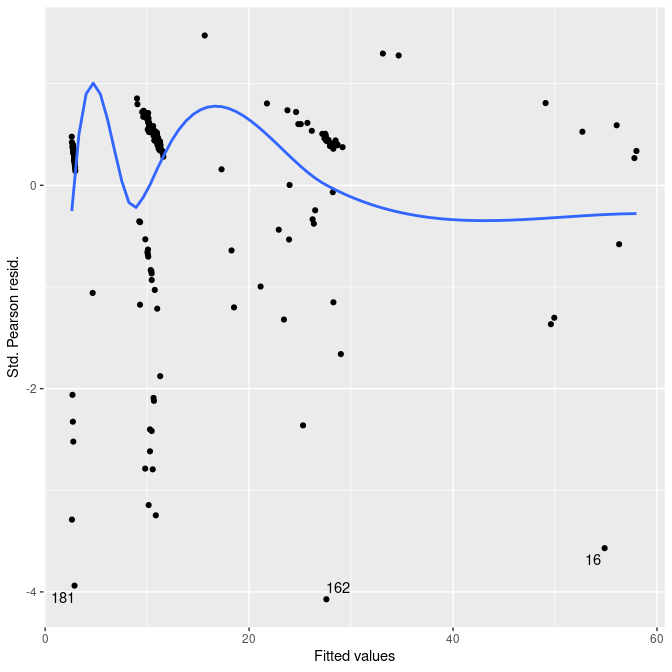##
## []
## Warning: Removed 9 rows containing non-finite values (stat_smooth()).
## Warning: Removed 9 rows containing missing values (geom_point()).
## Warning: Removed 9 rows containing missing values (geom_text_repel()).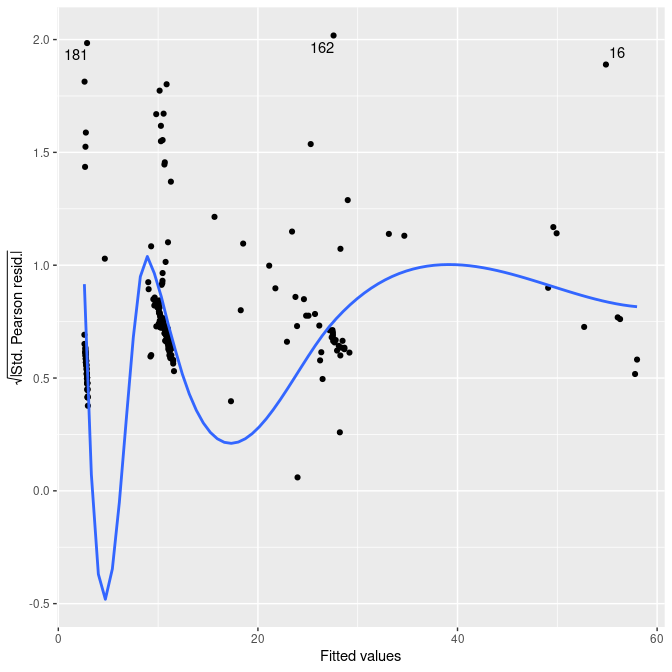##
## []
## Warning: Removed 9 rows containing non-finite values (stat_smooth()).
## Warning: Removed 9 rows containing missing values (geom_point()).
## Warning: Removed 9 rows containing missing values (geom_text_repel()).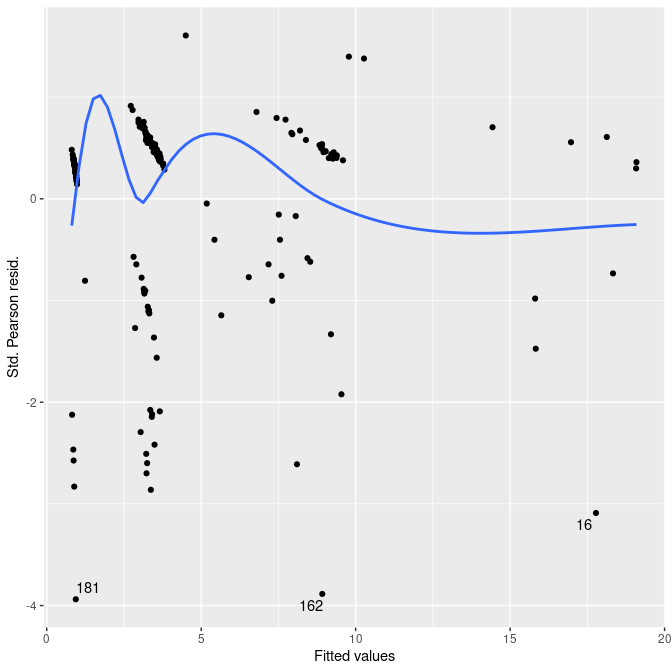##
## []
## Warning: Removed 9 rows containing non-finite values (stat_smooth()).
## Warning: Removed 9 rows containing missing values (geom_point()).
## Warning: Removed 9 rows containing missing values (geom_text_repel()).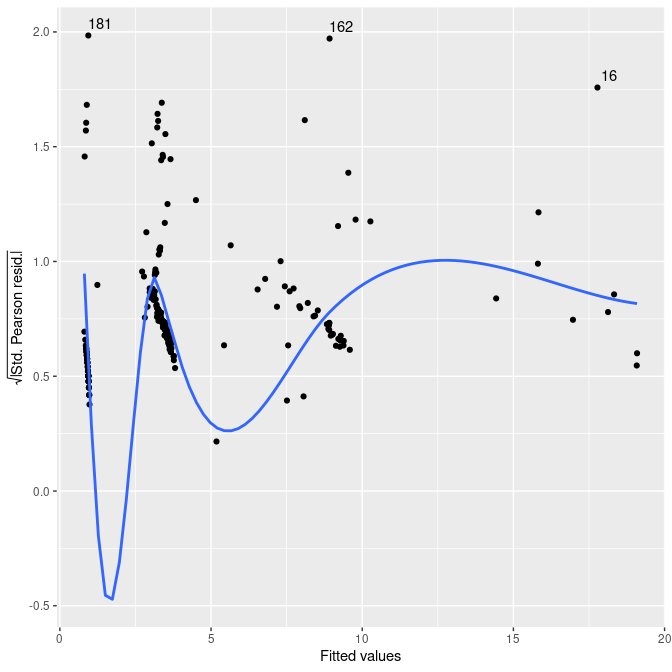The plots don’t look unreasonable.

Also make plots of residuals vs. square root of fitted and vs. network size:

autoplot(gof.wd, against=sqrt(.fitted))
## []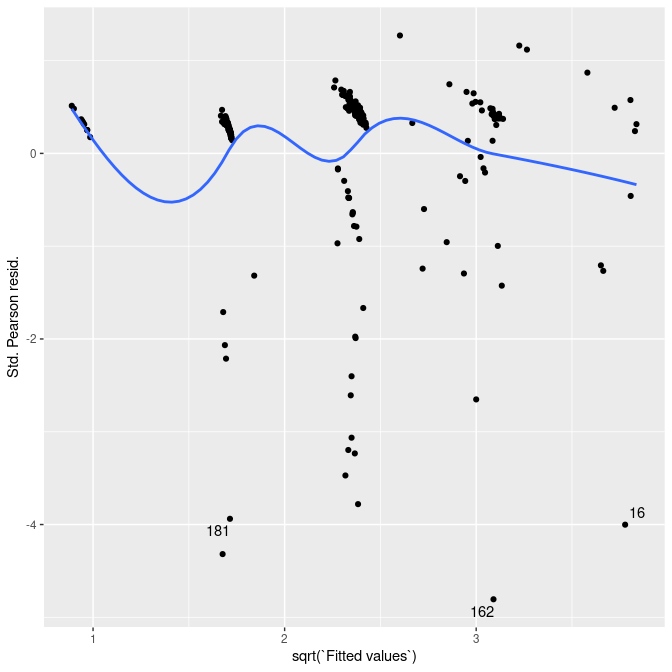##
## []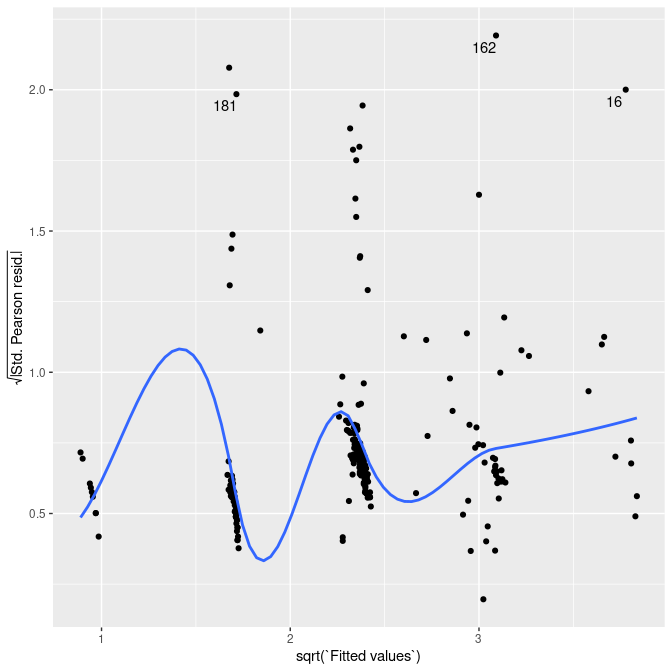##
## []
## Warning: Removed 9 rows containing non-finite values (stat_smooth()).
## Warning: Removed 9 rows containing missing values (geom_point()).
## Warning: Removed 9 rows containing missing values (geom_text_repel()).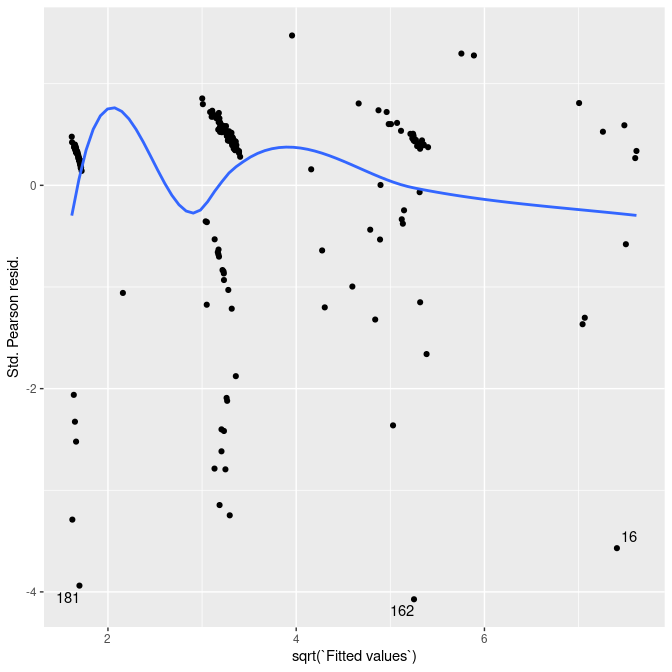##
## []
## Warning: Removed 9 rows containing non-finite values (stat_smooth()).
## Warning: Removed 9 rows containing missing values (geom_point()).
## Warning: Removed 9 rows containing missing values (geom_text_repel()).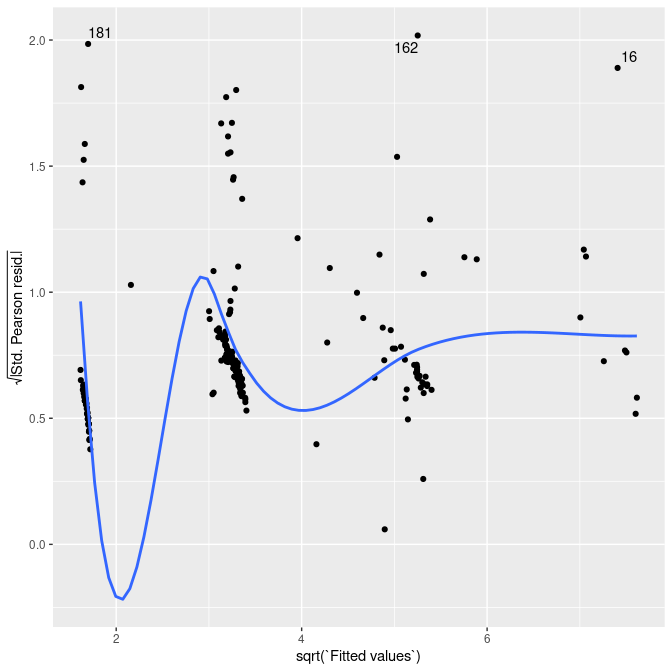##
## []
## Warning: Removed 9 rows containing non-finite values (stat_smooth()).
## Warning: Removed 9 rows containing missing values (geom_point()).
## Warning: Removed 9 rows containing missing values (geom_text_repel()).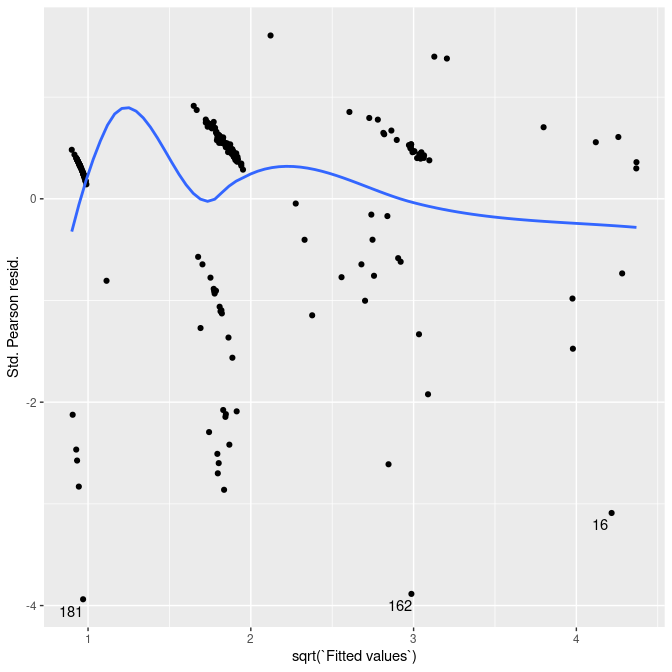##
## []
## Warning: Removed 9 rows containing non-finite values (stat_smooth()).
## Warning: Removed 9 rows containing missing values (geom_point()).
## Warning: Removed 9 rows containing missing values (geom_text_repel()).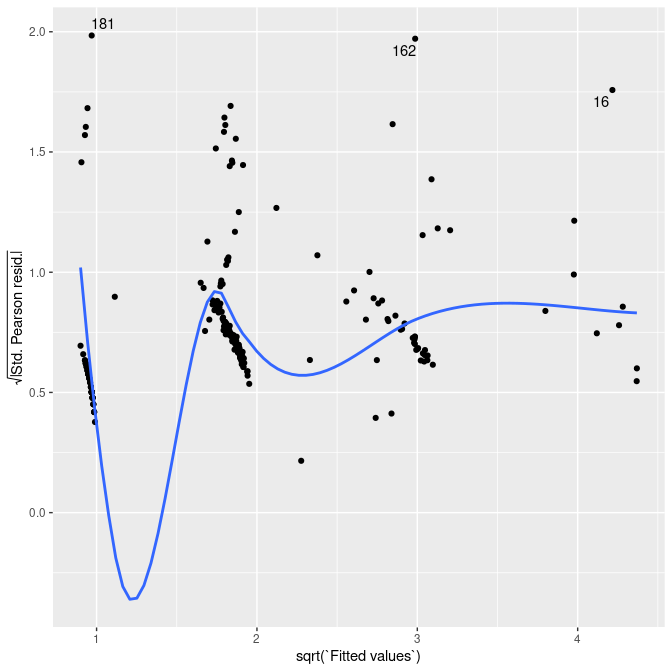autoplot(gof.wd, against=ordered(n))
## []
## Warning in simpleLoess(y, x, w, span, degree = degree, parametric = parametric, : pseudoinverse used at 0.975
## Warning in simpleLoess(y, x, w, span, degree = degree, parametric = parametric, : neighborhood radius 2.025
## Warning in simpleLoess(y, x, w, span, degree = degree, parametric = parametric, : reciprocal condition number 1.1489e-16
## Warning in simpleLoess(y, x, w, span, degree = degree, parametric = parametric, : There are other near singularities as well. 1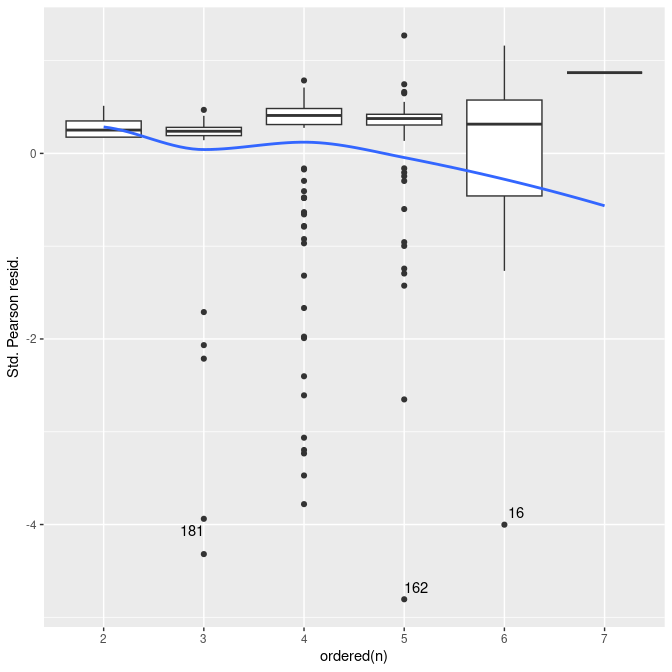##
## []
## Warning in simpleLoess(y, x, w, span, degree = degree, parametric = parametric, : pseudoinverse used at 0.975
## Warning in simpleLoess(y, x, w, span, degree = degree, parametric = parametric, : neighborhood radius 2.025
## Warning in simpleLoess(y, x, w, span, degree = degree, parametric = parametric, : reciprocal condition number 1.1489e-16
## Warning in simpleLoess(y, x, w, span, degree = degree, parametric = parametric, : There are other near singularities as well. 1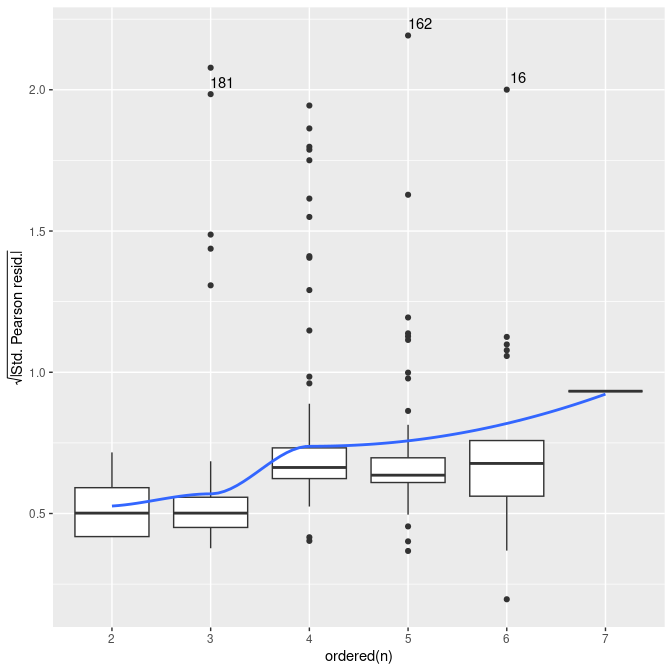##
## []
## Warning: Removed 9 rows containing non-finite values (stat_boxplot()).
## Warning: Removed 9 rows containing non-finite values (stat_smooth()).
## Warning in simpleLoess(y, x, w, span, degree = degree, parametric = parametric, : pseudoinverse used at 1.98
## Warning in simpleLoess(y, x, w, span, degree = degree, parametric = parametric, : neighborhood radius 1.02
## Warning in simpleLoess(y, x, w, span, degree = degree, parametric = parametric, : reciprocal condition number 0
## Warning in simpleLoess(y, x, w, span, degree = degree, parametric = parametric, : There are other near singularities as well. 1
## Warning: Removed 9 rows containing missing values (geom_text_repel()).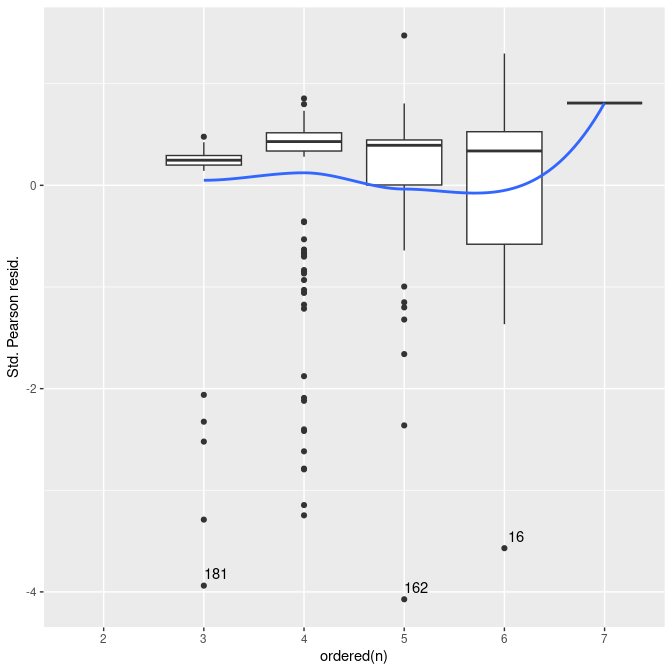##
## []
## Warning: Removed 9 rows containing non-finite values (stat_boxplot()).
## Warning: Removed 9 rows containing non-finite values (stat_smooth()).
## Warning in simpleLoess(y, x, w, span, degree = degree, parametric = parametric, : pseudoinverse used at 1.98
## Warning in simpleLoess(y, x, w, span, degree = degree, parametric = parametric, : neighborhood radius 1.02
## Warning in simpleLoess(y, x, w, span, degree = degree, parametric = parametric, : reciprocal condition number 0
## Warning in simpleLoess(y, x, w, span, degree = degree, parametric = parametric, : There are other near singularities as well. 1
## Warning: Removed 9 rows containing missing values (geom_text_repel()).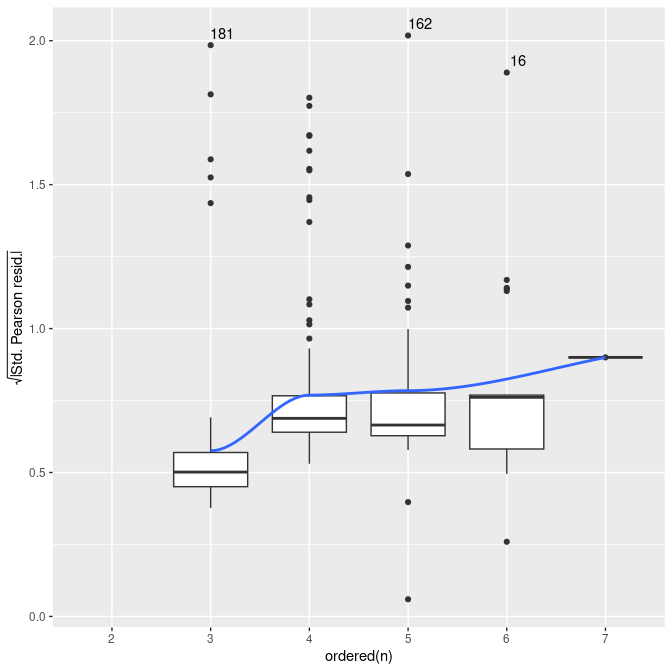##
## []
## Warning: Removed 9 rows containing non-finite values (stat_boxplot()).
## Warning: Removed 9 rows containing non-finite values (stat_smooth()).
## Warning in simpleLoess(y, x, w, span, degree = degree, parametric = parametric, : pseudoinverse used at 1.98
## Warning in simpleLoess(y, x, w, span, degree = degree, parametric = parametric, : neighborhood radius 1.02
## Warning in simpleLoess(y, x, w, span, degree = degree, parametric = parametric, : reciprocal condition number 0
## Warning in simpleLoess(y, x, w, span, degree = degree, parametric = parametric, : There are other near singularities as well. 1
## Warning: Removed 9 rows containing missing values (geom_text_repel()).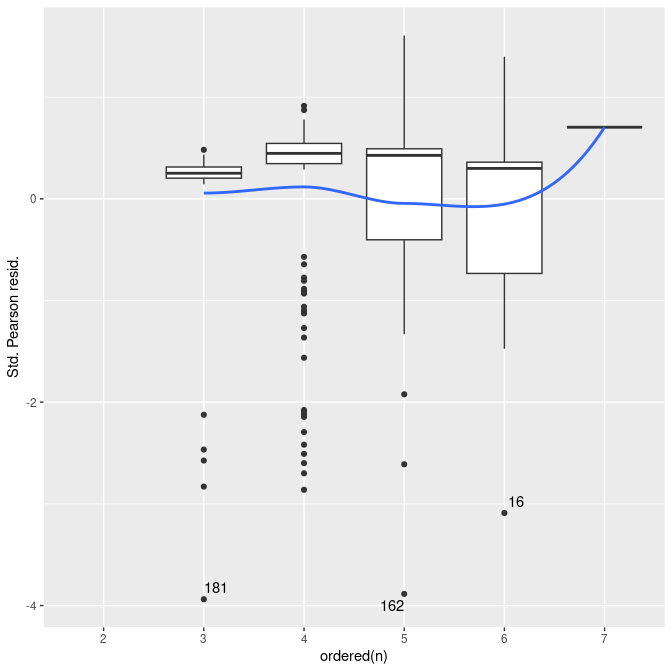##
## []
## Warning: Removed 9 rows containing non-finite values (stat_boxplot()).
## Warning: Removed 9 rows containing non-finite values (stat_smooth()).
## Warning in simpleLoess(y, x, w, span, degree = degree, parametric = parametric, : pseudoinverse used at 1.98
## Warning in simpleLoess(y, x, w, span, degree = degree, parametric = parametric, : neighborhood radius 1.02
## Warning in simpleLoess(y, x, w, span, degree = degree, parametric = parametric, : reciprocal condition number 0
## Warning in simpleLoess(y, x, w, span, degree = degree, parametric = parametric, : There are other near singularities as well. 1
## Warning: Removed 9 rows containing missing values (geom_text_repel()).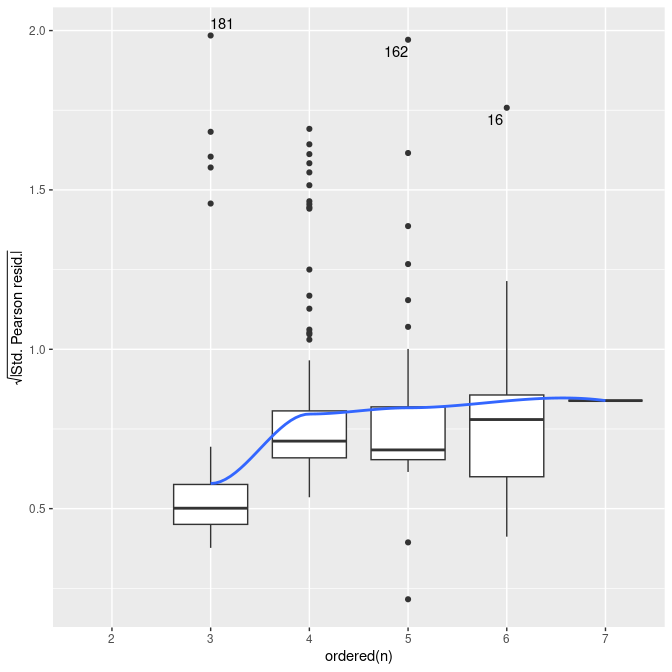It looks like network-size effects are probably accounted for.